Here we are providing Class 12 Maths Important Extra Questions and Answers Chapter 9 Differential Equations. Class 12 Maths Important Questions are the best resource for students which helps in Class 12 board exams.

## Class 12 Maths Chapter 9 Important Extra Questions Differential Equations

### Differential Equations Important Extra Questions Very Short Answer Type

Question 1.
Find the order and the degree of the differential equation: $$x^{2} \frac{d^{2} y}{d x^{2}}=\left[1+\left(\frac{d y}{d x}\right)^{2}\right]^{4}$$
(Delhi 2019)
Solution:
Here, order = 2 and degree = 1.

Question 2
Determine the order and the degree of the differential equation:$$\left(\frac{d y}{d x}\right)^{3}+2 y \frac{d^{2} y}{d x^{2}}=0$$ (C.B.S.E. 2019 C)
Solution:
Order = 2 and Degree = 1.

Question 3.
Form the differential equation representing the family of curves: y = b (x + a), where « and b are arbitrary constants. (C.B.S.E. 2019 C)
Solution:
Wehave:y= b(x + a) …(1)
Diff. w.r.t. x, b.
Again diff. w.r.t. x, $$\frac{d^{2} y}{d x^{2}}$$ = 0,
which is the reqd. differential equation.

Question 4.
Write the general solution of differential equation:
$$\frac{d y}{d x}$$ = ex+y (C.B.S.E. Sample Paper 2019-20)
Solution:
We have: $$\frac{d y}{d x}$$ = ex+y
⇒ e-y dy = ex dx [Variables Separable
Integrating, $$\int e^{-y} d y+c=\int e^{x} d x$$
⇒ – e-y + c = ex
⇒ ex + e-y = c.

Question 5.
Find the integrating factor of the differential equation:
y$$\frac{d y}{d x}$$ – 2x = y3e-y
Solution:
The given equation can be written as.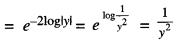Question 6.
Form the differential equation representing the family of curves y = a sin (3x – b), where a and b are arbitrary constants. (C.B.S.E. 2019C)
Solution:
We have: y – a sin (3x – b) …(1)
Diff. W.r.t y $$\frac{d y}{d x}$$ = a cos (3x – b) .3
= 3a cos (3x – b)
$$\frac{d^{2} y}{d x^{2}}$$ = -3a sin (3x – b) 3
= -9a sin (3x – b)
= -9y [Using (1)]
$$\frac{d^{2} y}{d x^{2}}$$ + 9y = 0,m
which in the reqd. differential equation.

### Differential Equations Important Extra Questions Short Answer Type

Question 1.
Determine the order and the degree of the differential equation:
$$\left(\frac{d y}{d x}\right)^{3}+2 y \frac{d^{2} y}{d x^{2}}=0$$ (Outside Delhi 2019C)
Solution:
Order = 2 and Degree = 1.

Question 2.
Form the differential equation representing the family of curves: y = e2x (a + bx), where ‘a’ and ‘h’ are arbitray constants. (Delhi 2019)
Solution:
We have : y = e2x (a + bx) …(1)
Diff. w.r.t. x, $$\frac{d y}{d x}$$ = e2x (b) + 2e2x (a + bx)
⇒ $$\frac{d y}{d x}$$ = be2x + 2y ………….. (2)
Again diff. w.r.t. x,
$$\frac{d^{2} y}{d x^{2}}$$ = 2be2x + 22x
$$\frac{d^{2} y}{d x^{2}}$$ = 2($$\frac{d y}{d x}$$ – 2y) + $$\frac{d y}{d x}$$
[Using (2)]
Hence, $$\frac{d^{2} y}{d x^{2}}$$ -4 $$\frac{d y}{d x}$$ + 4y = 0, which is the reqd. differential equation.

Question 3.
Solve the following differentia equation:
$$\frac{d y}{d x}$$ + y = cos x – sin x (Outside Delhi 2019)
Solution:
The given differential equation is :
$$\frac{d y}{d x}$$ + y = cos x – sin x dx Linear Equation
∴ I.F. = e∫1dx = ex
The solution is :
y.ex = ∫ (cos x — sin x) ex dx + C
⇒ y.ex = ex cos x + C
or y = cos x + C e-x

Question 4.
Solve the following differential equation :
$$\frac{d x}{d y}$$ + x = (tan y + sec2y). (Outside Delhi 2019 C)
Solution:
The given differential equation is :
$$\frac{d x}{d y}$$ + x = (tany + sec2y).
Linear Equation
∵ I.F. = Jldy = ey
∴ The solution is :
x. ey = ∫ ey (tan y + sec2 y)dy + c
⇒ x. ey = ey tan y + c
= x = tan y + c e-y, which is the reqd. solution.

### Differential Equations Important Extra Questions Long Answer Type 1

Question 1.
Solve the differential equation
(x2 – y2)dx + 2xydy = 0 (C.B.S.E. 2018)
Solution: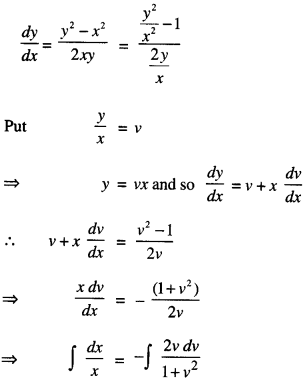log x = -log (1 + v2) + log C
x(1 + v2) = C
x(1 + $$\frac{y^{2}}{x^{2}}$$) = C
x2 + y2 = C.

Question 2.
Find the particular solution of the differential equation (1 + x2)$$\frac{d y}{d x}$$ + 2xy = $$\frac{1}{1+x^{2}}$$, given that y = 0 when x = 1(C.B.S.E. 2018 C)
Solution: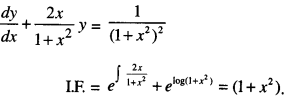Solution is y( 1 + x2) = $$\int \frac{1}{1+x^{2}} d x$$
= tan-1 x + C
When y = 0,x = 1,
then 0 = $$\frac{\pi}{4}$$ + C
C = $$\frac{\pi}{4}$$
∴ y(1 + x2) = tan -1 x – $$\frac{\pi}{4}$$
i.e, y = $$\frac{\tan ^{-1} x}{1+x^{2}}-\frac{\pi}{4\left(1+x^{2}\right)}$$

Question 3.
Find the differential equation representing the family of curves y = aebx + 5, where ‘a’and ‘A’are arbitrary constants. {C.B.S.E. 2018)
Solution:
We have: y = aebx + 5 + 5 …(1)
Diff. w.r.t. x, $$\frac{d y}{d x}$$ = aebx + 5. (b)
$$\frac{d y}{d x}$$ = dy ……(2) [Using (1)]]
Again diff. w.r.t x.,
$$\frac{d^{2} y}{d x^{2}}=b \frac{d y}{d x}$$ ………(3)

Dividing (3) by (2),which is the required differential equation.

Question 4.
Find the particular solution of the differential equation x dx – yey $$\sqrt{1+x^{2}}$$ dy = 0, given that y = 1 when x = 0. (C.B.S.E. 2019 C)
Solution:
The given differential equation is: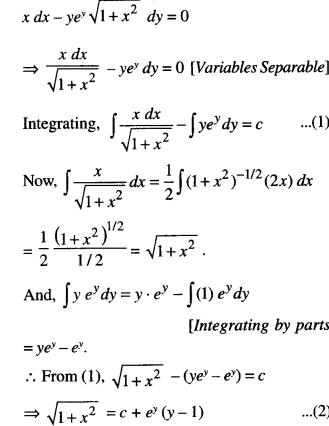When x = 0,y = 1, ∴ 1 = c + c(0) ⇒ c = 1.
Putting in (2), $$\sqrt{1+x^{2}}$$ = 1 + ey(y -1),
which is the reqd. particular solution.

Question 5.
Obtain the differential equation of the family of circles, which touch the x-axis at the origin.   (N.C.E.R.T.; C.B.S.E. Sample Paper 2018)
Solution:
Let (0, α) be the centre of any member of the family of circles.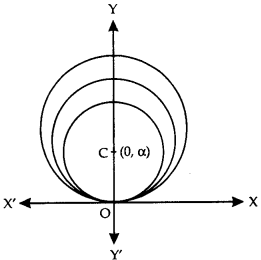Then the equation of the family of circles is : x2 + (y-α)2 = α2
⇒ x2 + y2 – 2αy = 0 …(1)
Diff. w.r.t. x, 2x + 2y $$\frac{d y}{d x}$$ 2α $$\frac{d y}{d x}$$ = 0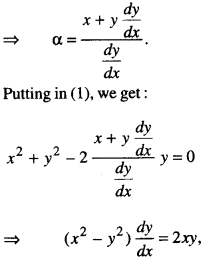which is the required differential equation.

Question 6.
Obtain the differential equation representing the family of parabolas having vertex at the origin and axis along the positive direction of x-axis. (N.C.E.R.T.)
Solution:
Let S (a, 0) be the focus of any member of the family of parabolas.
Then the equation of the family of curves is y2 = 4 ax …………. (1)
Diff. w.r.t. x, 2y$$\frac{d y}{d x}$$ = 4a ……………. (2)
Using (2) in (1), we get:
y2 = (2y$$\frac{d y}{d x}$$)xy2 – 2xy$$\frac{d y}{d x}$$ = 0
which is the required differential equation.

Question 7.
Find the general solution of the differential equation:
(tan2x + 2 tanx + 5) $$\frac{d y}{d x}$$ = 2 (1 + tanx) sec2 x
Solution:
We have : (tan2 x + 2 tan x + 5)$$\frac{d y}{d x}$$
= 2 (1 + tanx) sec2x
Integrating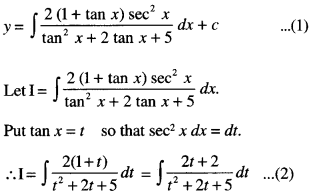Put t2 + 2t + 5 = z
so that (2t+ 2) dt = dz.
∴ From (2),
1 = $$\int \frac{d z}{z}$$ = log| z | = log |t2 + 2t + 5|
= log |tan2 x| + 2 tan x + 5|
From (1), y = log |tan2 x| + 2 tan x + 5| + c,
which is the required general solution.

Question 8.
Solve the differential equation:
(x + 1) $$\frac{d y}{d x}$$ = 2e-y – 1 ; y(0) = 0. (C.B.S.E. 2019)
Solution:
The given equation can be written as :
$$\frac{d y}{2 e^{-y}-1}=\frac{d x}{x+1}$$
| Variables Separable

Integrating, $$\int \frac{e^{y} d y}{2-e^{y}}=\int \frac{d x}{x+1}$$
⇒ – log |2 – ey| + log |C| = log |x + 1|
⇒ (2 – ey) (x + 1) = C.
When x = 0, y = 0, then C = 1.
Hence, the solution is (2 – ey) (x + 1) = 1.

Question 9.
Solve: (a) (i) $$\frac{d y}{d x}$$ = 1 + x + y + xy
(ii) xyy’ = 1 + x + y + xy. (N.C.E.R.T.)
(b) Find the particular solution of the differential dy
equation $$\frac{d y}{d x}$$ = 1 + x + y + xy, given that y = 0 when x = 1. (A.I.C.B.S.E. 2014)
Solution:
(a), (i) The given equation is :
$$\frac{d y}{d x}$$ = 1 + x + y + xy
$$\frac{d y}{d x}$$ = (1 +x) (1 +y)
$$\frac{d}{1+y}$$ = (1 + x)dx.
|Variables Separable

Integrating,
$$\int \frac{d y}{1+y}$$ = ∫(1 + x)dx + c
⇒ log |1 + y | = x + $$\frac { 1 }{ 2 }$$ x2 + c,
which is the required solution.

(ii) The given equation is xyy’ = 1 + x + y + xy
⇒ xy$$\int \frac{d y}{1+y}$$ = (1 + x)(1 + y)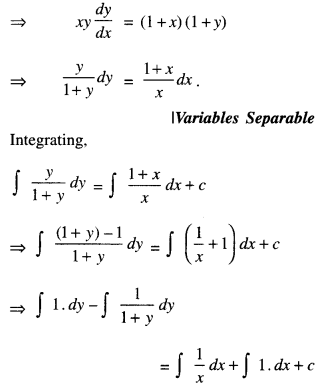= y – log|1 + y| = log|x|+ x + c
= x + log |x (1 + y)|+ c,
which is the required solution.

(b) From part (a) (i),
Iog|1+y| = x + $$\frac { 1 }{ 2 }$$x2 + c …(1)
When x = 1, y = 0, then:
log |1+0|= 1 + $$\frac { 1 }{ 2 }$$(1)2 + c
log 1 = 1 + $$\frac { 1 }{ 2 }$$ + c
0 = $$\frac { 3 }{ 2 }$$ + c
c = $$-\frac { 3 }{ 2 }$$
Putting in (1), log |1 + y| = x + $$\frac { 1 }{ 2 }$$x2 – $$\frac { 3 }{ 2 }$$
which is the required particular solution.

Question 10.
Solve the differential equation:
$$\frac{d y}{d x}$$ = 1 + x2 + y2 + x2y2
given thaty = 1 when x = 0. (Outside Delhi 2019)
Solution:
The given equation is $$\frac{d y}{d x}$$ = 1 + x2 + y2 + x2y2
⇒ $$\frac{d y}{d x}$$ = (1+x2)(1 +y2)
⇒ $$\frac{d y}{1+y^{2}}$$ (x2 + 1) dx
|Variables Separable

Integrating, ∫$$\frac{d y}{1+y^{2}}$$ = ∫(x2 + 1) dx + C
tan-1y = $$\frac{x^{3}}{3}$$ + x + C ………. (1)
Where x = 0,y = 1,
∴ tan-1 (1) = C
C = $$\frac{\pi}{4}$$
Putting in (1),
tan-1 y = $$\frac{x^{3}}{3}+x+\frac{\pi}{4}$$
which is the reqd. particular solution.

Question 11.
Find the particular solution of the differential equation: ex tan y dx + (2 – ex) sec2 y dy = 0, given thaty = $$\frac{\pi}{4}$$ where x = 0.
Solution:
The given differential equation is: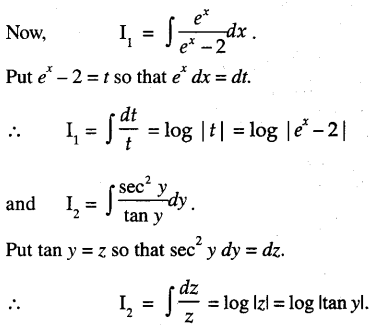∴ From (1),
log C + log |ex – 2| = log |tan y|
⇒ tany = C(ex – 2) …(2)
When x = 0, y = y = $$\frac{\pi}{4}$$
∴ 1 = C(1 – 2)
C = -1
Putting in (2),
tan y = 2 – ex
which is the required particular solution.

Question 12.
Find the particular solution of the following differential equation:
cosy dx + (1 + 2e-x) siny dy = 0; y(0) = $$\frac{\pi}{4}$$ .
(CBSE Sample Paper 2018 – 19)
Solution:
The given differential equation is:
cos y dx + (1 + 2e-x) sin ydy = 0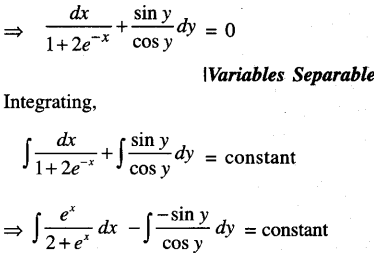log |ex| – log |cos y| = log|C|
ex + 2 = C cos y ….. (1)

Substituting, x = 0, y = $$=\frac{\pi}{4}$$ in(1),we get:
1 + 2 = C cos $$=\frac{\pi}{4}$$
C = $$\frac{3}{1 / \sqrt{2}}$$
C = 3√2
Putting in (1),
ex + 2 = 3√2 cos y
which is the required particular solution.

Question 13.
Find the particular solution of the following differential equation:
(x + 1) $$\frac{d y}{d x}$$ = 2e-y – 1 ; y = 0 when x = 0. (C.B.S.E. 2012)
Solution:
The given equation is (x + 1) $$\frac{d y}{d x}$$ = 2e-y – 1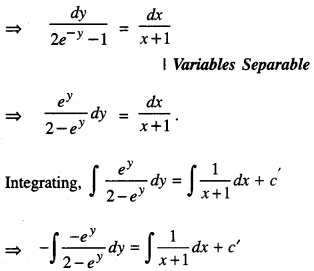-log|2 – ey| = log|x + 1| + log|c|,
where c’ = log |c|
⇒ log $$\left|\frac{1}{2-e^{y}}\right|$$ = log |c(x + 1)|
⇒ $$\frac{1}{2-e^{y}}$$ = c(x + 1)
When x = 0, y = 0;
$$\frac{1}{2-e^{0}}$$ = c(0 + 1)
⇒ c = $$\frac{1}{2-1}=\frac{1}{1}$$ = 1

Putting (1)
$$\frac{1}{2-e^{y}}$$ = 1.(x + 1)
⇒ (x + 1)(2 – ey) = 1
which is the required particular solution.

Question 14.
Solve the differential equation:
(1 – y2) (1 + log x)dx + 2xy dy = 0, given that when x = 1 ,y = 0.
(C.B.S.E. 2016)
Solution:
The given equation is :
(1 – y2) (1 + log x)dx + 2xy dy = 0Putting in (1),
$$\frac{(1+\log x)^{2}}{2}$$ = log|1 – y2| + 1/2
(1 + log x)2 = 2 log|1 – y2| + 1
which is the required solution.

Question 15.
Solve the differential equation:
x cos x ($$\frac { y }{ x }$$) $$\frac{d y}{d x}$$ = y cos($$\frac { y }{ x }$$) = x (C.B.S.E. 2019 C)
Solution:
The given equation is:Put $$\frac { y }{ x }$$ = v i.e, y = vx so that $$\frac { dy }{ dx }$$ = v + x $$\frac { dv }{ dx }$$
∴ (1) becomes:
cos v (v + x$$\frac { dv }{ dx }$$) = vcosv + 1
= v + x $$\frac { dv }{ dx }$$ = v + $$\frac { 1 }{ cos v }$$
x $$\frac { dv }{ dx }$$ = $$\frac { 1 }{ cos v }$$
cos v dv = $$\frac { dx }{ x }$$
[Variables Seperable]

Integrating, ∫ cos v dv = ∫ $$\frac{d x}{ x}$$ + c
sin = log|x| + c
sin $$\frac{y}{ x}$$ = log|x| + c,
which is the reqd. solution.

Question 16.
Find the general solution of the differential equation: $$\frac{d x}{d y}=\frac{y \tan y-x \tan y-x y}{y \tan y}$$ (C.B.S.E. Sample Paper 2018-19)
Solution:
The given differential equation is :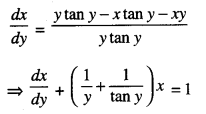|Linear Equation
Comparing with $$\frac{d x}{d y}$$ + Py = Q, we have :
‘P’ = $$\frac{1}{y}+\frac{1}{\tan y}$$ and ‘Q’ = 1

∴ I.F = $$e^{\int\left(\frac{1}{y}+\frac{1}{\tan y}\right) d y}=e^{\log y+\log \sin y}$$
elog(ysiny) = ysiny.
∴ The solution is
x x I.F. = ∫ (Q x I.F.)dy + C
⇒ x(y sin y) = ∫(1 x y sin y)dy + C
⇒ xy sin y = ∫ y sin y dy + C
⇒ xy sin y = y (- cos y) – ∫(1) -cos y)dy + C

(Integrating by Parts)
⇒ xy sin y = – y cos y + ∫ cos ydy + C
⇒ xy sin y = – y cos y + sin y + C
⇒ x = $$\frac{\sin y-y \cos y+C}{y \sin y}$$
which is the required general solution.

Question 17.
Solve the differential equation :
xdy – ydx = $$\sqrt{x^{2}+y^{2}}$$ dx , given that y = 0 when x = 1. (C.B.S.E. 2019)
Solution:
The given equation can be written as :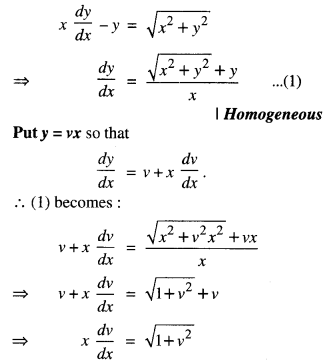where x = 1, y = 0
∴ 0 + $$\sqrt{1+0}$$ = c(1)
⇒ c = 1
Putting in (2), y + $$\sqrt{x^{2}+y^{2}}$$ = x2,
which is the reqd. solutions.

Question 18.
Solve the following differential equation:
$$x y \log \left(\frac{y}{x}\right) d x+\left(y^{2}-x^{2} \log \left(\frac{y}{x}\right)\right) d y=0$$ (C.B.S.E. 2010 C)
Solution:
The given equation can be written as: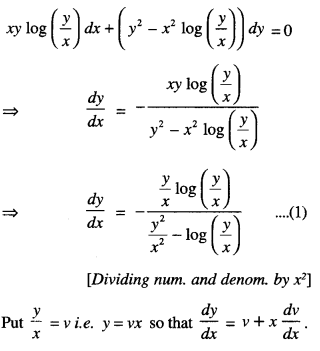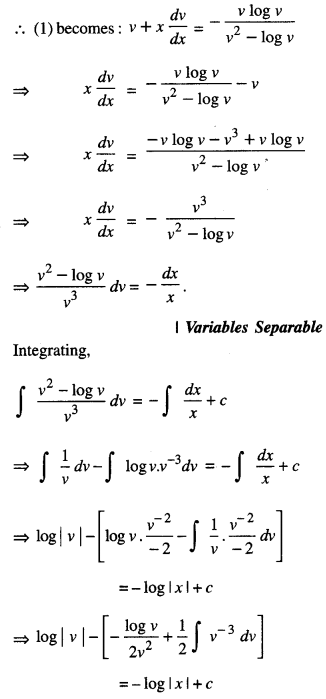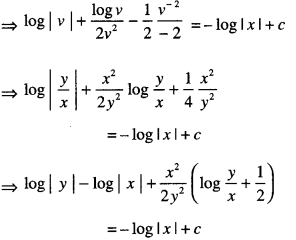Question 19.
Solve the differential equation:
xdy-y dx= $$\sqrt{x^{2}+y^{2}}$$ dx.
(iC.B.S.E. Sample Paper 2019)
Solution:
The given differential equation is:Put y = vx, so that $$\frac{d y}{d x}$$ = dv + x $$\frac{d v}{d x}$$
(1) becomes:⇒ y+ $$\sqrt{x^{2}+y^{2}}$$ = cx2,
which is the reqd. solution.

Question 20.
Prove that x2 – y2 = c(x2 + y2)2 is the general solution of the differential equation: (x3 – 3xy2)dx = (y3 – 3x2y)dy, where c is a parameter. (C.B.S.E. 2017)
Solution:
We have :(x3 – 3xy2) dx = (y3 – 3x2y)dy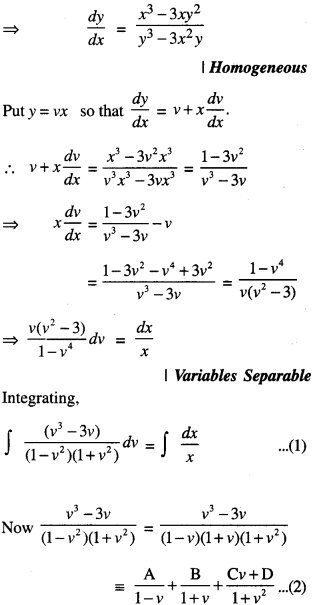∴ v3 – 3v = A(1 + v)(1 + v2) + B(1 – v) (1 + v2) + (Cv + D) (1 – v2).
Putting v= 1,
1-3 = A(2) (2)
⇒ A = -1/2

Putiing v = -1, -1 + 3 = B(2)(1 + 1)
⇒ B = 1/2

Putting v = 0
⇒ 0 = A(1)(1) + B(1)(1) + D(1)
⇒ 0 = $$-\frac{1}{2}+\frac{1}{2}$$ + D
D = 0
Comparing coeff. of v3, 1 = A – B – C
⇒ C = A – B – 1 = $$-\frac{1}{2}-\frac{1}{2}$$ – 1 = -2
∴ From (2),Squaring , x2 – y2 = c’2(x2+ y2)2
⇒ x2 – y2 = c’2 (x2 + y2 )2
where c’2 = c,
which is the required solution.

Question 21.
Show that the differential equation:
2yex/y dx + (y – 2x ex/y) dy = 0 is homogeneous and find the particular solution, given that x = 0 when y = 1. (C.B.S.E. 2013)
Solution:
(i) The given equation is :
2yex/y dx + (y – 2x ex/y) dy = 0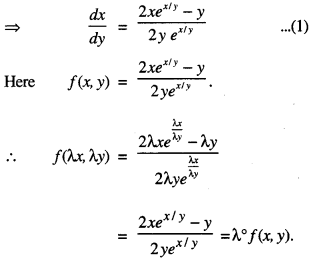Thus f(x, y) is homogeneous function of degree zero.⇒ 2ev = – log |y| + c
⇒ ex/y = -log|y| + c …………….(2)

Now x = 0 when y = 1,
∴ 2(1) = -log |1| + c
⇒ 2 = – log 1 + c
⇒ 2 = —0 + c
⇒ c = 2.

Putting in (2),
2ex/y = – log |y|+ 2,
which is the required solution.

Question 22.
Solve the differential equation:
(1 + x2)$$\frac{d y}{d x}$$ + 2xy – 4x2 =0, subject to the initial condition y (0) = 0. (C.B.S.E. 2019)
Solution:
The given equation can be written as :Multiplying (1) by (1 + x2), we get:
(1 + x2)$$\frac{d y}{d x}$$ + 2xy = 4x2 dx
⇒ $$\frac{d}{d x}$$ y.(1 + x2) = 4x2

Integrating, y.(1 + x2) = ∫ 4x2 dx = $$\frac{4 x^{3}}{3}$$ + C
⇒ y(1 + x2) = $$\frac{4}{3}$$ x2 + C …(2)
When x = 0, y = 0,
∴ C = 0 + C
⇒ C = 0.
ry 4 T
Putting in (2), y(1 + x2) = $$\frac{4}{3}$$ x3 , which is the required solution.

Question 23.
Solve the differential equation :
$$\frac{d y}{d x}-\frac{2 x}{1+x^{2}} y$$ = x2 + 2 (C.B.S.E. 2019)
Solution:
The given equation is :Hence, y = (1 + x2) (x + tan-1 x + C).

Question 24.
Find the general solution of the following differential equation:
(1 + y2) + (x – etan-1y)$$\frac{d y}{d x}$$ (C.B.S.E. 2016)
Solution:
The given equation is:Putting xeetan-1y = $$\frac{1}{2}$$e2tan-1y + c
x = $$\frac{1}{2}$$etan-1y + c
which is the required solution.

Question 25.
(i) Solve the differential equation:
(tan-1 y – x)dy = (1 + y2) dx. (N.C.E.R.T.; C.B.S.E. 2015)
(ii) Find the particular solution when x = 0, y = 0. (A.I.C.B.S.E. 2013)
Solution:
(i) The given equation can be written as :
$$\frac{d x}{d y}+\frac{x}{1+y^{2}}=\frac{\tan ^{-1} y}{1+y^{2}}$$ …… (1)
Comparing with $$\frac{d x}{d y}$$+ Px = Q , we have: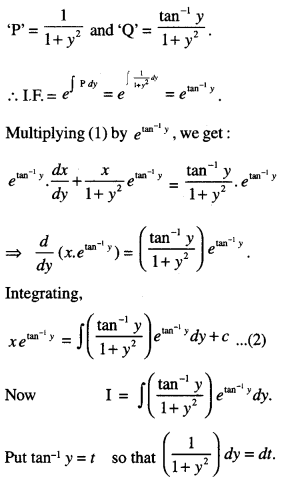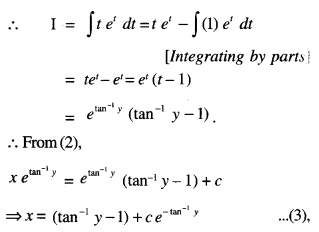which is the required solution.

(ii) When
x – 0, y = 0.
0 = (tan-10 – 1) + ce-tan-10,
⇒ 0 – (0 – 1) + ce-0
⇒ 0 = – 1 + c
⇒ c = 1.
Putting in (3),
x = (tan-1 y – 1) + e-tan-1y,
which is the required particular solution.